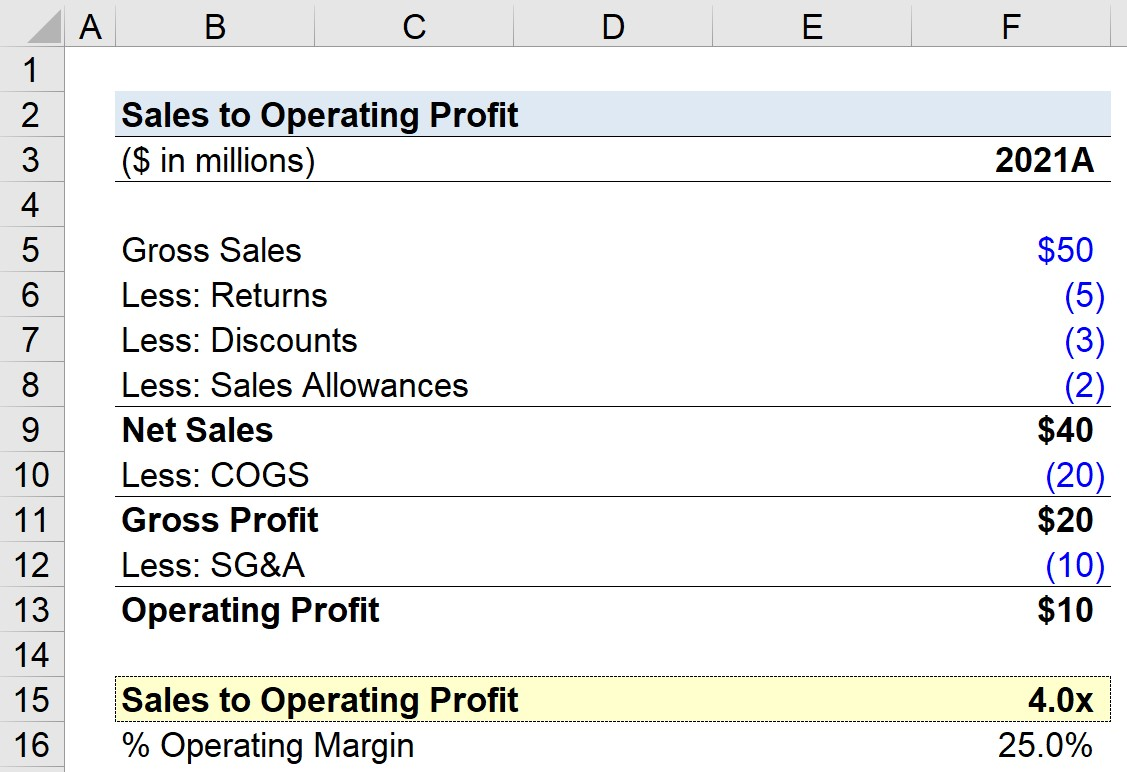Welcome to Wall Street Prep! Use code at checkout for 15% off.# Sales to Operating Profit

Guide to Understanding the Operating Profit to Sales Ratio## How to Calculate Sales to Operating Profit Ratio

The sales to operating profit ratio compares a company’s net sales to its operating profit.

Simply put, the sales to operating profit ratio is the approximate amount of revenue that a company must produce in order to generate a dollar in operating profit.

The metric is primarily used to set internal revenue targets so that the company can improve its operating profitability.

## Sales to Operating Profit Ratio Formula

The formula for calculating the sales to operating profit ratio is as follows.

###### Sales to Operating Profit Formula
• Sales to Operating Profit = Net Sales ÷ Operating Profit

The inputs can be calculated using the following equations.

• Net Sales = Gross Sales – Returns – Discounts – Sales Allowances
• Operating Profit = Net Sales – COGS – Operating Expenses

By flipping the formula around, we’re left with the operating margin metric.

###### Operating Margin Formula
• Operating Margin = Operating Profit ÷ Net Sales

The operating margin shows how much of one dollar of revenue generated by a company flows down to the operating income (EBIT) line item.

## Sales to Operating Profit Ratio — Excel Model Template

We’ll now move to a modeling exercise, which you can access by filling out the form below.Submitting...

## Sales to Operating Profit Ratio Calculation Example

Suppose a company generated \$50 million in gross sales in 2021, but there was a total of \$10 million in deductions related to returns, discounts, and sales allowances.

Further, the company incurred \$20 million in COGS and \$10 million in SG&A.

• Gross Profit = \$40 million – \$20 million = \$20 million
• Operating Profit = \$20 million – \$10 million = \$10 million

Given those assumptions, our company’s gross profit is \$20 million while its operating profit is \$10 million.

Financials 2021A
Gross Sales \$50 million
Less: Returns (\$5 million)
Less: Discounts (\$3 million)
Less: Sales Allowances (\$2 million)
Net Sales \$40 million
Less: COGS (20 million)
Gross Profit \$20 million
Less: SG&A (10 million)
Operating Profit \$10 million

By dividing the \$10 million in operating profit by the \$40 million in net sales, the operating margin comes out to 25%.

• Operating Margin = \$10 million ÷ \$40 million = 25%

In the final part of our exercise, we’ll calculate our company’s sales to operating profit ratio using the formula below, which results in a ratio of 4.0x.

• Sales to Operating Profit = \$40 million ÷ \$10 million = 4.0x

The 4.0x sales to operating profit ratio means that the company must generate \$4.00 in revenue for its operating profit to be \$1.00.Step-by-Step Online Course

### Everything You Need To Master Financial Modeling

Enroll in The Premium Package: Learn Financial Statement Modeling, DCF, M&A, LBO and Comps. The same training program used at top investment banks.

Inline Feedbacks